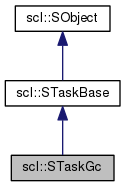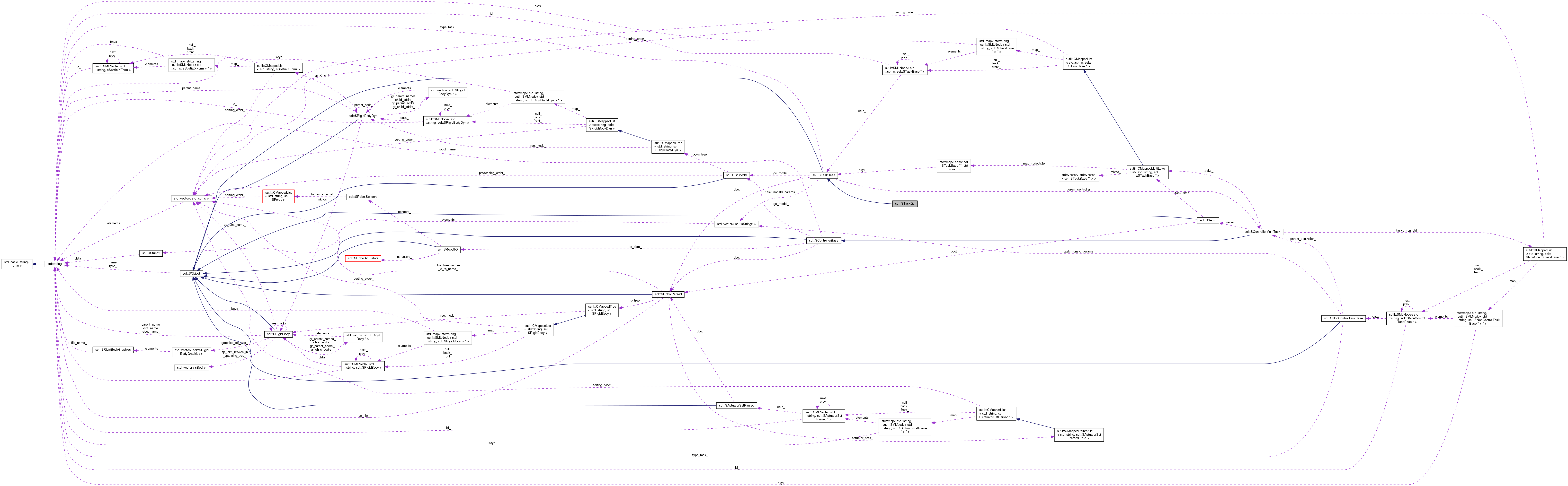SCL  1.0 Standard Control Library : Control, dynamics, physics, and simulation[legend][legend]

Public Member Functions

bool init (const std::string &arg_name, const std::string &arg_type, const sUInt arg_priority, const scl::sUInt arg_task_dof, const SRobotParsed *arg_robot_ds, const SGcModel *arg_gc_model, const Eigen::VectorXd &arg_kp, const Eigen::VectorXd &arg_kv, const Eigen::VectorXd &arg_ka, const Eigen::VectorXd &arg_ki, const Eigen::VectorXd &arg_ftask_max, const Eigen::VectorXd &arg_ftask_min, const std::vector< scl::sString2 > &arg_nonstd_params)

virtual const std::string & getType () const

virtual const std::string & getName () const

virtual bool hasBeenInit () const

Public Attributes

Eigen::VectorXd q_

Eigen::VectorXd dq_

Eigen::VectorXd ddq_

Eigen::VectorXd q_goal_

Eigen::VectorXd dq_goal_

Eigen::VectorXd ddq_goal_

sFloat spatial_resolution_

sBool gravity_enabled_

scl::sBool has_been_activated_

scl::sBool has_control_null_space_

sUInt priority_

const SRobotParsedrobot_

const SGcModelgc_model_

Eigen::MatrixXd J_

Eigen::MatrixXd J_6_

Eigen::MatrixXd J_dyn_inv_

Eigen::MatrixXd null_space_

Eigen::VectorXd force_gc_

Eigen::MatrixXd range_space_

Eigen::VectorXd kp_

Eigen::VectorXd kv_

Eigen::VectorXd ka_

Eigen::VectorXd ki_

Eigen::VectorXd shared_data_

std::string name_

sBool has_been_init_

Protected Attributes

std::string type_

Constructor & Destructor Documentation

Default constructor sets stuff to S_NULL

virtual

Default destructor does nothing

Member Function Documentation

 virtual const std::string& scl::SObject::getName ( ) const
inlinevirtualinherited

Get the object's type

 virtual const std::string& scl::SObject::getType ( ) const
inlinevirtualinherited

Get the object's type

 virtual bool scl::SObject::hasBeenInit ( ) const
inlinevirtualinherited

Get the object's type

 bool scl::STaskBase::init ( const std::string & arg_name, const std::string & arg_type, const sUInt arg_priority, const scl::sUInt arg_task_dof, const SRobotParsed * arg_robot_ds, const SGcModel * arg_gc_model, const Eigen::VectorXd & arg_kp, const Eigen::VectorXd & arg_kv, const Eigen::VectorXd & arg_ka, const Eigen::VectorXd & arg_ki, const Eigen::VectorXd & arg_ftask_max, const Eigen::VectorXd & arg_ftask_min, const std::vector< scl::sString2 > & arg_nonstd_params )
inherited

Initialization function

NOTE : This function also activates the task.

Parameters
 arg_task_dof 0 task dof means a gc task. Ie. full dofs arg_nonstd_params These are ignored during STaskBase initialization. However, subclasses may choose to use them and/or require various values
virtual
1. Initializes the task specific data members.

inherited

Sets the parent controller

Member Data Documentation

inherited

inherited

Generalized coordinate forces (usually joint torques) : Computed by : the task-servo = J' * f Used by : the robot-servo to calculate overall generalized coordinate force

inherited

Task space forces Computed by : the task-servo Used by : the task-servo to compute the force_gc_

inherited

Task-space centrifugal/coriolis force vector Controls cc forces in a subspace of the entire gc space so that the operational point experience no cc forces. Other points, however, do.

inherited

Task-space gravity force vector. Controls gravity in a subspace of the entire gc space so that the operational point is massless. Other points experience gravity.

inherited

Upper and lower limits of task-space forces (Why separate : Consider muscles which can only pull, not push)

inherited

Robot generalized coordinate model

inherited

Whether the task is active or inactive Default = false. True after init()

 sBool scl::SObject::has_been_init_
inherited

Whether the object is ready for use

inherited

Is control task. This variable controls whether the controller computes remaining null spaces for lower level tasks or not. If it is true, the lower level task null spaces are not computed, potentially improving performance substantially.

Settings: True : Task dof < Robot dof [Default] False : Task dof == Robot dof [Must enable manually in init]

inherited

The computed task space Jacobian matrices. Used for velocities. Note that we compute the generalized inverse (the Jacobian is usually not full rank) without inverting the jacobian itself.

inherited

Gains (scalar if same for different dimensions; vector if different for different dimensions) kp = position, kv = velocity, ka = acceleration ki = integrated position error (to fix steady-state errors).

Computed by : None. These are constants. Used by : The task-servo to calculate task forces

inherited

 std::string scl::SObject::name_
inherited

The object's name

inherited

The task's null space. Used to compute the range space of lower priority tasks.

inherited

The parent controller

inherited

The task's priority. Important to determine its range space. Lower is better. 0 is best. -1 is NULL.

inherited

Range space of the task : Computed by : the robot-servo to calculate overall generalized coordinate force Used by : the robot-servo to calculate overall generalized coordinate force

inherited

Robot model. Not a const because it could use the branching representation's iterator

inherited

This may be used by any shared module in scl that wants to communicate arbitrary information to a STaskBase subclass

inherited

A set of additional options that may be used by users to initialize this specific task. These typically go above and beyond the standard options.

 std::string scl::SObject::type_
protectedinherited

The object's type. Should only be set by the constructor